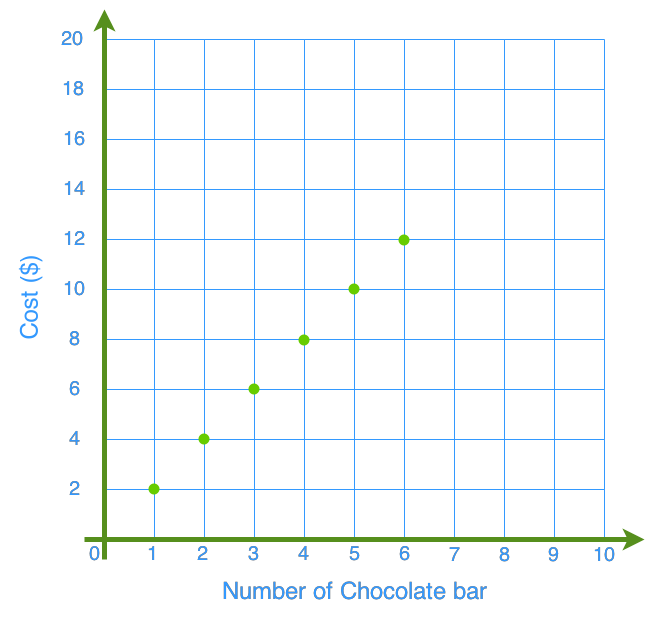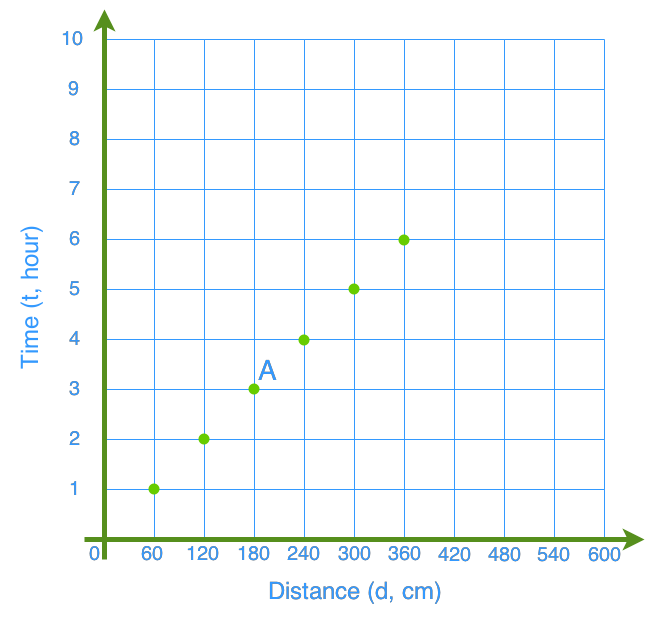# Understanding graphs of linear relationships

### Understanding graphs of linear relationships

Without understanding and analyzing, graphs of linear relationships are just lines and dots. In this lesson, we will learn the skills to analyze the graphs and get useful information from them.
Basic Concepts: Ratios, Proportions

#### Lessons

• 1.
The graph below shows the cost of chocolate bars..
a)
Describe the patterns represented on the graph.

b)
Create a table of values from the graph.

c)
From the graph, estimate the cost of 12 chocolate bars.

d)
It is reasonable to add a point between the ones on the graph? Why or why not?

• 2.
The graph shows the distance a car travels..
a)
What does (180, 3) represent?

b)
What is the speed of the car at point A?

c)
Is the graph showing a linear relationship? Why or why not?

d)
It is reasonable to add a point between the ones on the graph? Explain.